# Chapter 3 Calculations with Chemical Formulas and Equations

• Slides: 62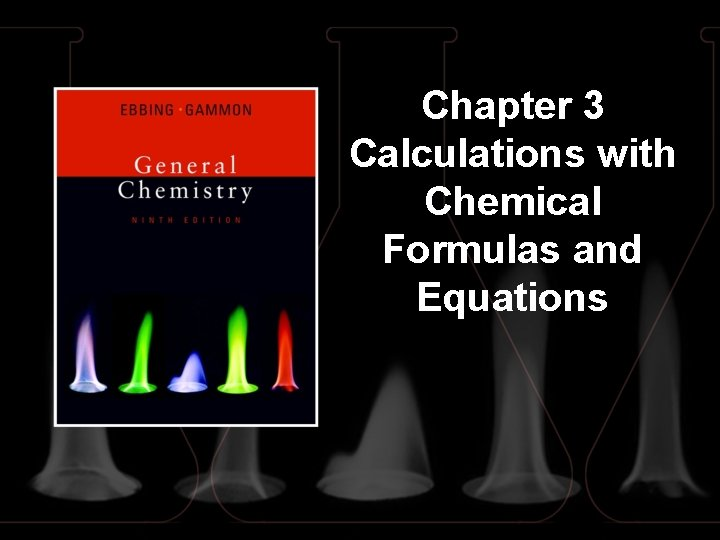Chapter 3 Calculations with Chemical Formulas and Equations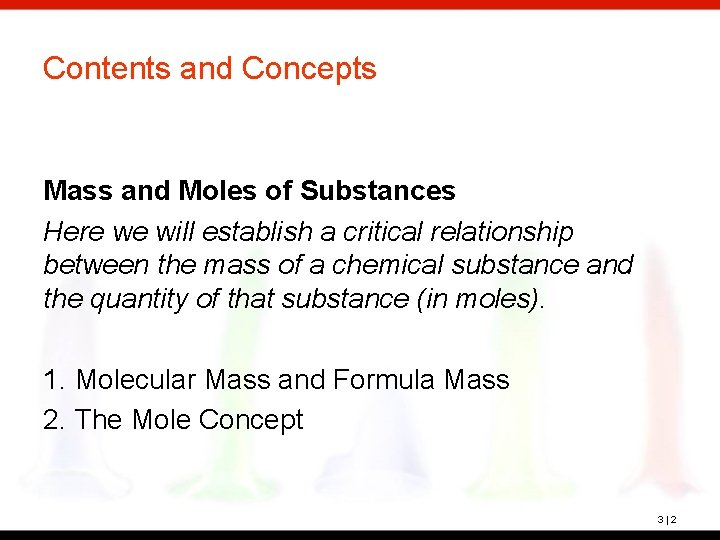Contents and Concepts Mass and Moles of Substances Here we will establish a critical relationship between the mass of a chemical substance and the quantity of that substance (in moles). 1. Molecular Mass and Formula Mass 2. The Mole Concept 3|2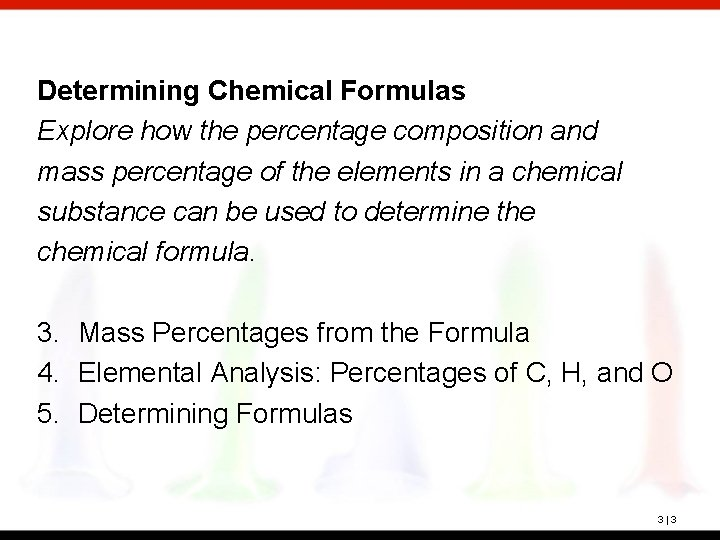Determining Chemical Formulas Explore how the percentage composition and mass percentage of the elements in a chemical substance can be used to determine the chemical formula. 3. Mass Percentages from the Formula 4. Elemental Analysis: Percentages of C, H, and O 5. Determining Formulas 3|3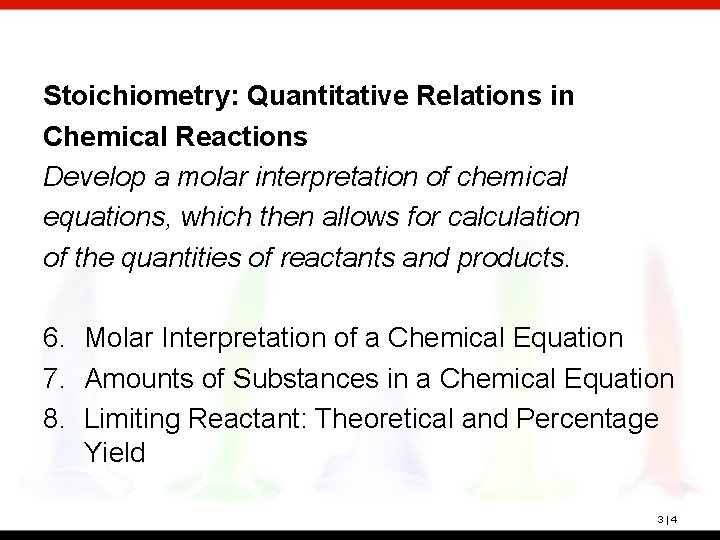Stoichiometry: Quantitative Relations in Chemical Reactions Develop a molar interpretation of chemical equations, which then allows for calculation of the quantities of reactants and products. 6. Molar Interpretation of a Chemical Equation 7. Amounts of Substances in a Chemical Equation 8. Limiting Reactant: Theoretical and Percentage Yield 3|4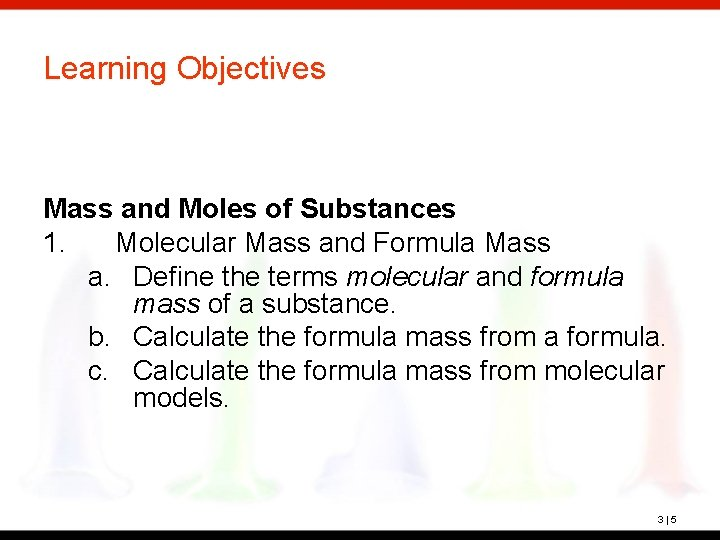Learning Objectives Mass and Moles of Substances 1. Molecular Mass and Formula Mass a. Define the terms molecular and formula mass of a substance. b. Calculate the formula mass from a formula. c. Calculate the formula mass from molecular models. 3|5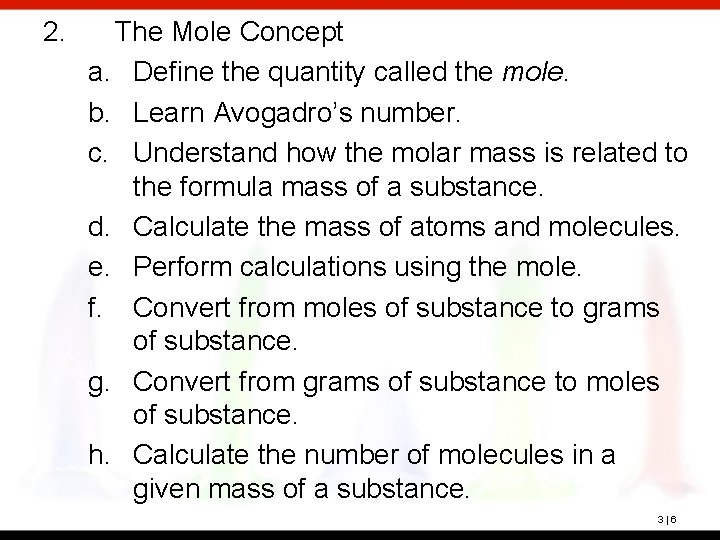2. The Mole Concept a. Define the quantity called the mole. b. Learn Avogadro’s number. c. Understand how the molar mass is related to the formula mass of a substance. d. Calculate the mass of atoms and molecules. e. Perform calculations using the mole. f. Convert from moles of substance to grams of substance. g. Convert from grams of substance to moles of substance. h. Calculate the number of molecules in a given mass of a substance. 3|6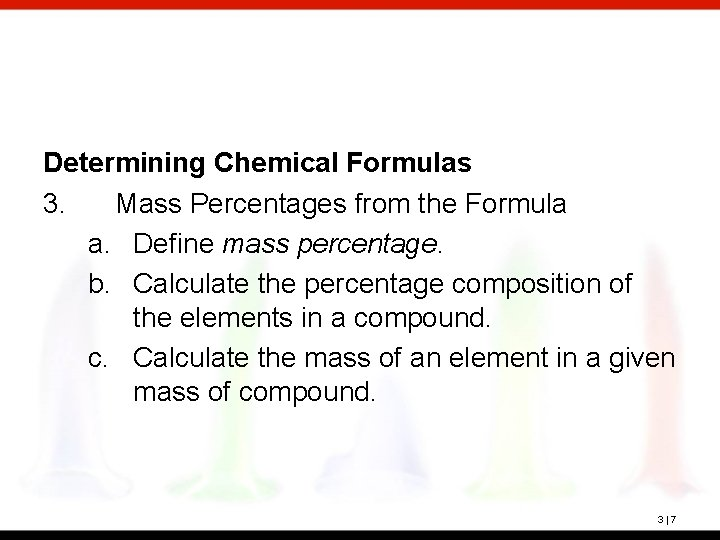Determining Chemical Formulas 3. Mass Percentages from the Formula a. Define mass percentage. b. Calculate the percentage composition of the elements in a compound. c. Calculate the mass of an element in a given mass of compound. 3|7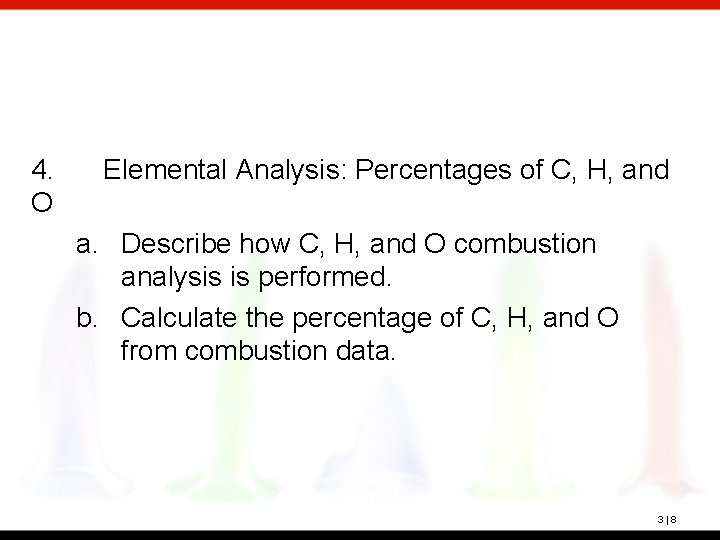4. O Elemental Analysis: Percentages of C, H, and a. Describe how C, H, and O combustion analysis is performed. b. Calculate the percentage of C, H, and O from combustion data. 3|8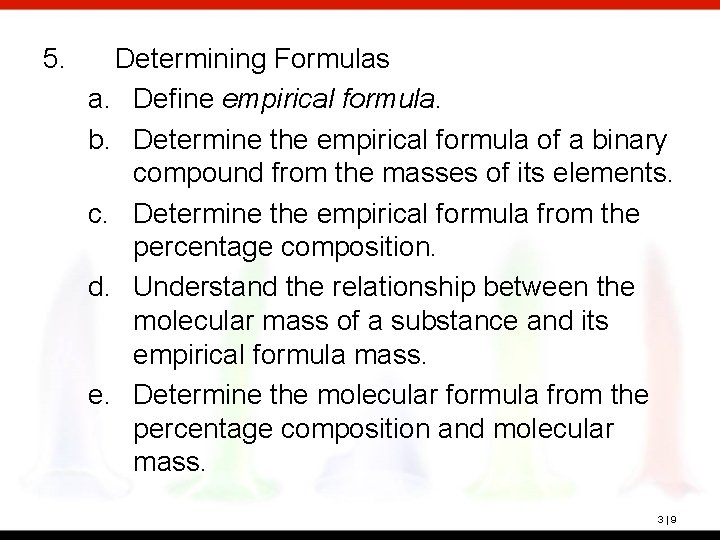5. Determining Formulas a. Define empirical formula. b. Determine the empirical formula of a binary compound from the masses of its elements. c. Determine the empirical formula from the percentage composition. d. Understand the relationship between the molecular mass of a substance and its empirical formula mass. e. Determine the molecular formula from the percentage composition and molecular mass. 3|9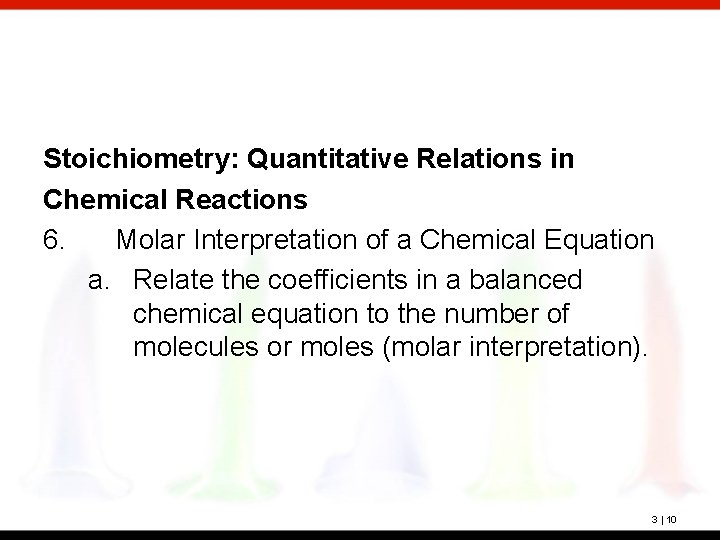Stoichiometry: Quantitative Relations in Chemical Reactions 6. Molar Interpretation of a Chemical Equation a. Relate the coefficients in a balanced chemical equation to the number of molecules or moles (molar interpretation). 3 | 10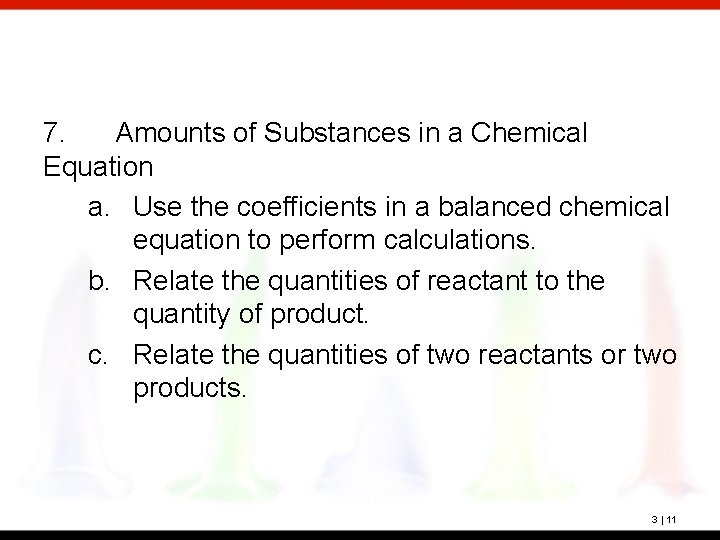7. Amounts of Substances in a Chemical Equation a. Use the coefficients in a balanced chemical equation to perform calculations. b. Relate the quantities of reactant to the quantity of product. c. Relate the quantities of two reactants or two products. 3 | 11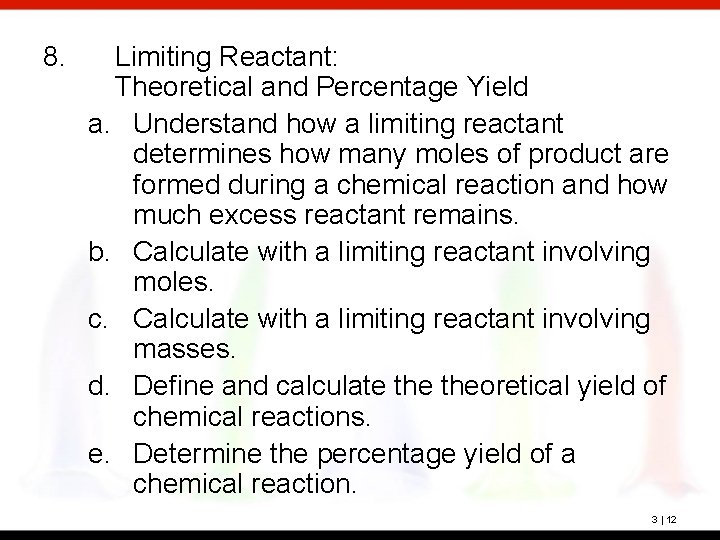8. Limiting Reactant: Theoretical and Percentage Yield a. Understand how a limiting reactant determines how many moles of product are formed during a chemical reaction and how much excess reactant remains. b. Calculate with a limiting reactant involving moles. c. Calculate with a limiting reactant involving masses. d. Define and calculate theoretical yield of chemical reactions. e. Determine the percentage yield of a chemical reaction. 3 | 12Molecular Mass The sum of the atomic masses of all the atoms in a molecule of the substance. Formula Mass The sum of the atomic masses of all atoms in a formula unit of the compound, whether molecular or not. 3 | 13? Calculate the formula weight of the following compounds from their formulas. Report your answers to three significant figures. calcium hydroxide, Ca(OH)2 methylamine, CH 3 NH 2 3 | 14Ca(OH)2 1 Ca 1(40. 08) = 40. 08 amu 2 O 2(16. 00) = 32. 00 amu 2 H 2(1. 008) = 2. 016 amu Total 74. 095 3 significant figures 74. 1 amu CH 3 NH 2 1 C 1(12. 01) = 12. 01 amu 1 N 1(14. 01) = 14. 01 amu 5 H 5(1. 008) = 5. 040 amu Total 31. 060 3 significant figures 31. 1 amu 3 | 15? What is the mass in grams of the nitric acid molecule, HNO 3? First, find the molar mass of HNO 3: 1 H 1(1. 008) = 1 N 1(14. 01) = 3 O 3(16. 00) = 1. 008 14. 01 48. 00 63. 018 (2 decimal places) 63. 02 g/mol 3 | 16Mole, mol The quantity of a given amount of substance that contains as many molecules or formula units as the number of atoms in exactly 12 g of carbon-12. Avogadro’s Number, NA The number of atoms in exactly 12 g of carbon-12 NA = 6. 02 × 1023 (to three significant figures). 3 | 17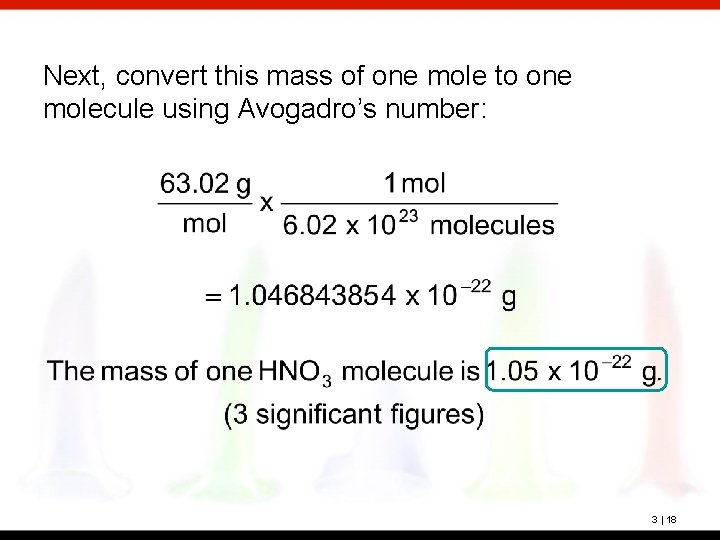Next, convert this mass of one mole to one molecule using Avogadro’s number: 3 | 18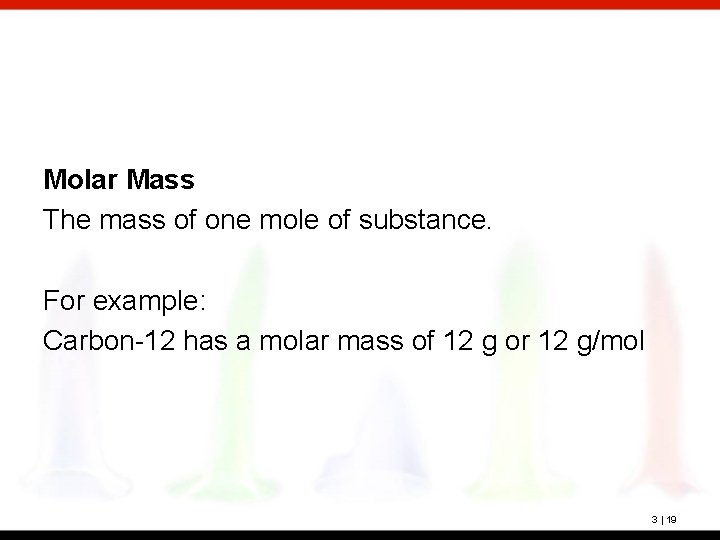Molar Mass The mass of one mole of substance. For example: Carbon-12 has a molar mass of 12 g or 12 g/mol 3 | 19? A sample of nitric acid, HNO 3, contains 0. 253 mol HNO 3. How many grams is this? First, find the molar mass of HNO 3: 1 H 1(1. 008) = 1 N 1(14. 01) = 3 O 3(16. 00) = 1. 008 14. 01 48. 00 63. 018 (2 decimal places) 63. 02 g/mol 3 | 20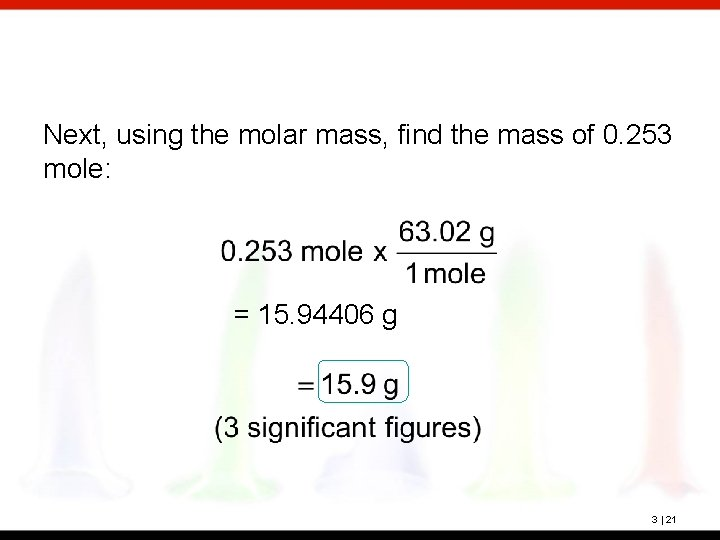Next, using the molar mass, find the mass of 0. 253 mole: = 15. 94406 g 3 | 21? Calcite is a mineral composed of calcium carbonate, Ca. CO 3. A sample of calcite composed of pure calcium carbonate weighs 23. 6 g. How many moles of calcium carbonate is this? First, find the molar mass of Ca. CO 3: 1 Ca 1(40. 08) = 1 C 1(12. 01) = 3 O 3(16. 00) = 40. 08 12. 01 48. 00 100. 09 2 decimal places 100. 09 g/mol 3 | 22Next, find the number of moles in 23. 6 g: 3 | 23? The average daily requirement of the essential amino acid leucine, C 6 H 14 O 2 N, is 2. 2 g for an adult. What is the average daily requirement of leucine in moles? First, find the molar mass of leucine: 6 C 2 O 1 N 14 H 6(12. 01) = 2(16. 00) = 1(14. 01) = 14(1. 008) = 72. 06 32. 00 14. 01 14. 112 132. 182 2 decimal places 132. 18 g/mol 3 | 24Next, find the number of moles in 2. 2 g: 3 | 25? The daily requirement of chromium in the human diet is 1. 0 × 10 -6 g. How many atoms of chromium does this represent? 3 | 26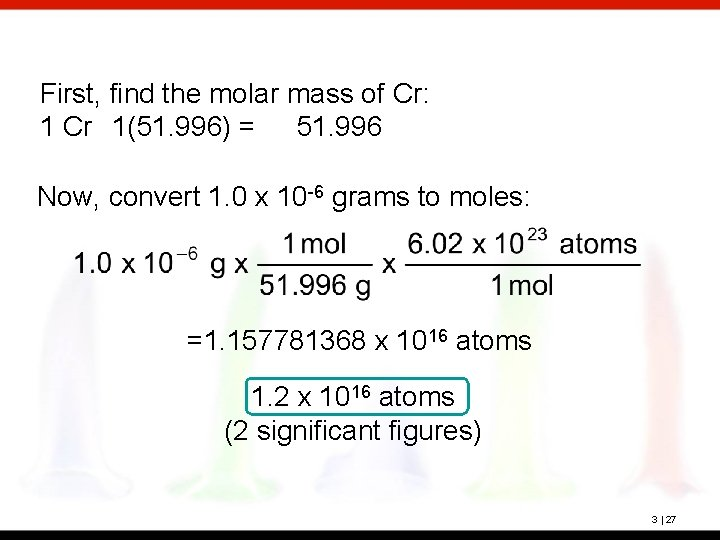First, find the molar mass of Cr: 1 Cr 1(51. 996) = 51. 996 Now, convert 1. 0 x 10 -6 grams to moles: =1. 157781368 x 1016 atoms 1. 2 x 1016 atoms (2 significant figures) 3 | 27? Lead(II) chromate, Pb. Cr. O 4, is used as a paint pigment (chrome yellow). What is the percentage composition of lead(II) chromate? First, find the molar mass of Pb. Cr. O 4: 1 Pb 1(207. 2) = 207. 2 1 Cr 1(51. 996) = 51. 996 4 O 4(16. 00) = 64. 00 323. 196 (1 decimal place) 323. 2 g/mol 3 | 28Now, convert each to percent composition: Check: 64. 11 + 16. 09 + 19. 80 = 100. 00 3 | 29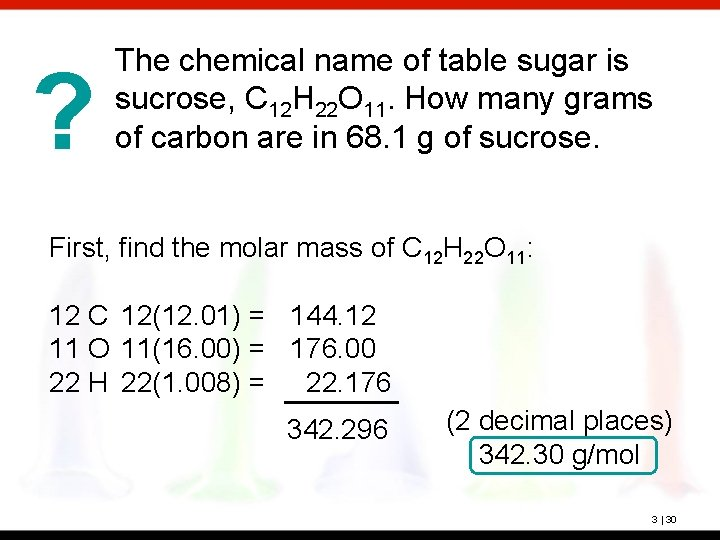? The chemical name of table sugar is sucrose, C 12 H 22 O 11. How many grams of carbon are in 68. 1 g of sucrose. First, find the molar mass of C 12 H 22 O 11: 12 C 12(12. 01) = 144. 12 11 O 11(16. 00) = 176. 00 22 H 22(1. 008) = 22. 176 342. 296 (2 decimal places) 342. 30 g/mol 3 | 30Now, find the mass of carbon in 61. 8 g sucrose: 3 | 31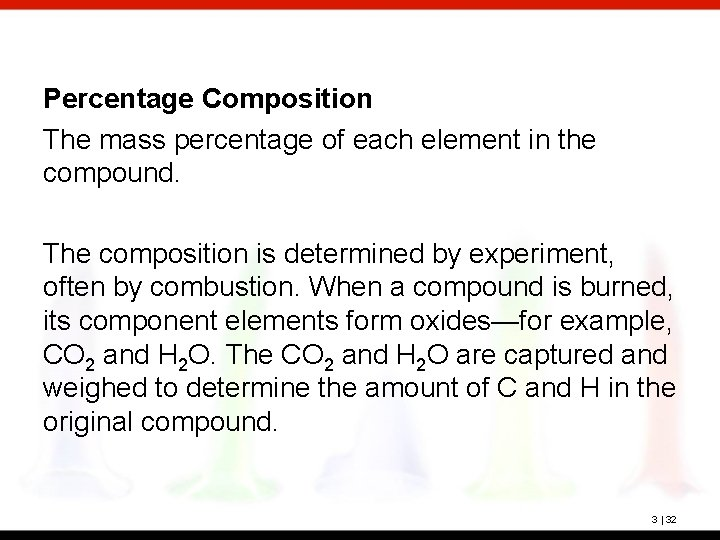Percentage Composition The mass percentage of each element in the compound. The composition is determined by experiment, often by combustion. When a compound is burned, its component elements form oxides—for example, CO 2 and H 2 O. The CO 2 and H 2 O are captured and weighed to determine the amount of C and H in the original compound. 3 | 32? Benzene is a liquid compound composed of carbon and hydrogen; it is used in the preparation of polystyrene plastic. A sample of benzene weighing 342 mg is burned in oxygen and forms 1156 mg of carbon dioxide. What is the percentage composition of benzene? 3 | 33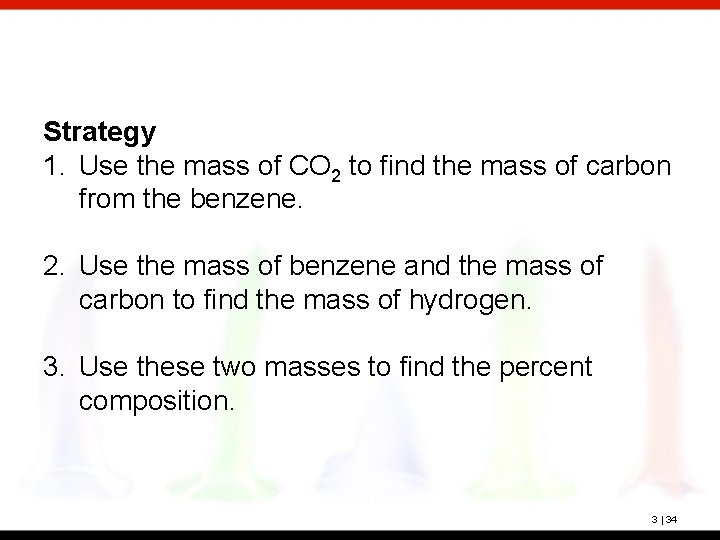Strategy 1. Use the mass of CO 2 to find the mass of carbon from the benzene. 2. Use the mass of benzene and the mass of carbon to find the mass of hydrogen. 3. Use these two masses to find the percent composition. 3 | 34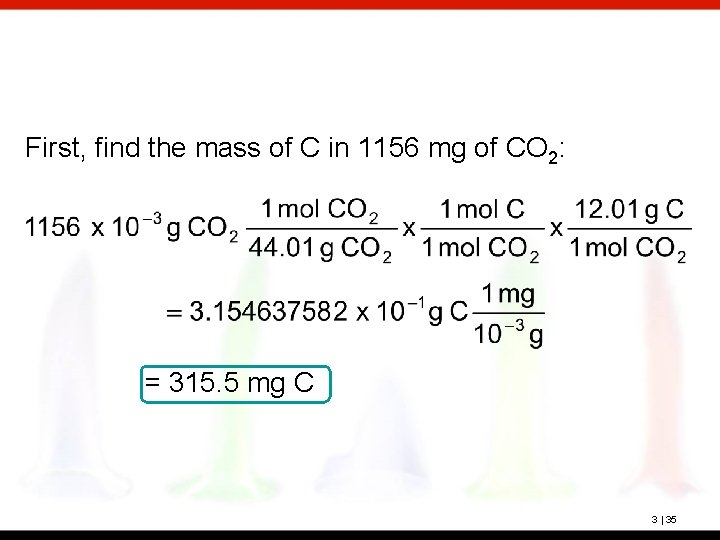First, find the mass of C in 1156 mg of CO 2: = 315. 5 mg C 3 | 35Next, find the mass of H in the benzene sample: 342 mg benzene -315. 5 mg C 26. 5 mg H (the decimal is not significant) Now, we can find the percentage composition: 3 | 36Empirical Formula (Simplest Formula) The formula of a substance written with the smallest integer subscripts. For example: The empirical formula for N 2 O 4 is NO 2. The empirical formula for H 2 O 2 is HO 3 | 37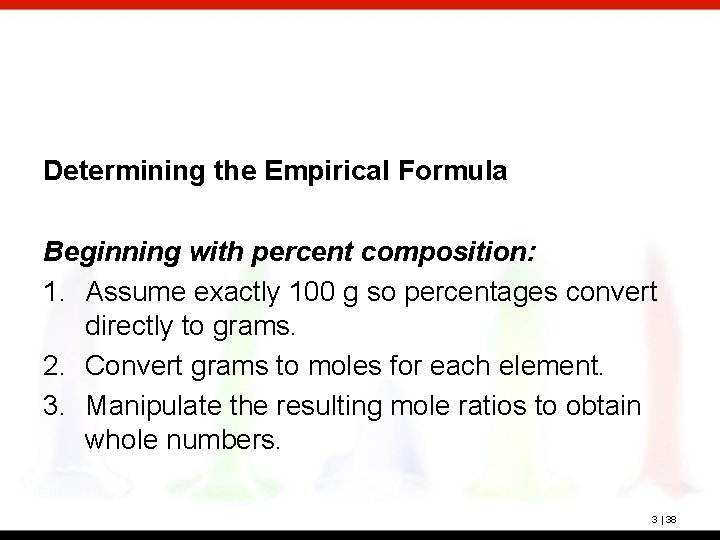Determining the Empirical Formula Beginning with percent composition: 1. Assume exactly 100 g so percentages convert directly to grams. 2. Convert grams to moles for each element. 3. Manipulate the resulting mole ratios to obtain whole numbers. 3 | 38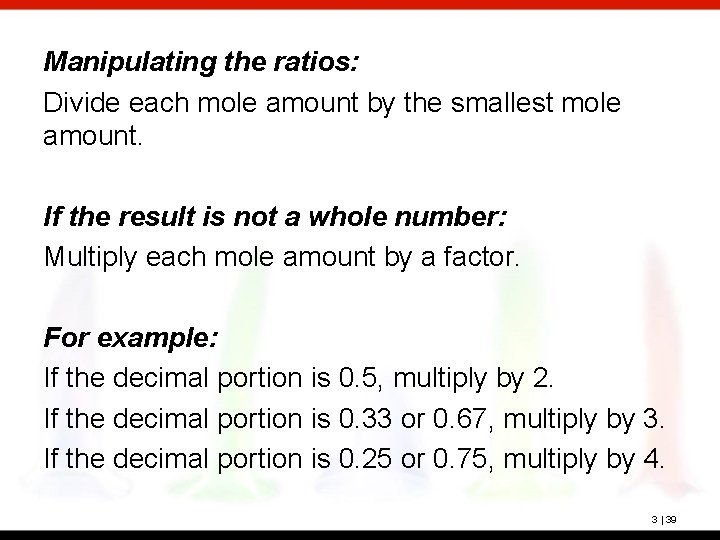Manipulating the ratios: Divide each mole amount by the smallest mole amount. If the result is not a whole number: Multiply each mole amount by a factor. For example: If the decimal portion is 0. 5, multiply by 2. If the decimal portion is 0. 33 or 0. 67, multiply by 3. If the decimal portion is 0. 25 or 0. 75, multiply by 4. 3 | 39? Benzene is composed of 92. 3% carbon and 7. 7% hydrogen. What is the empirical formula of benzene? Empirical formula: CH 3 | 40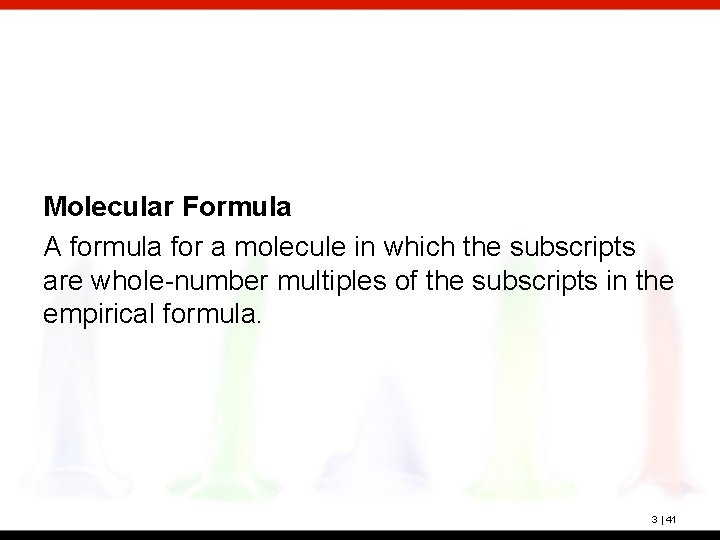Molecular Formula A formula for a molecule in which the subscripts are whole-number multiples of the subscripts in the empirical formula. 3 | 41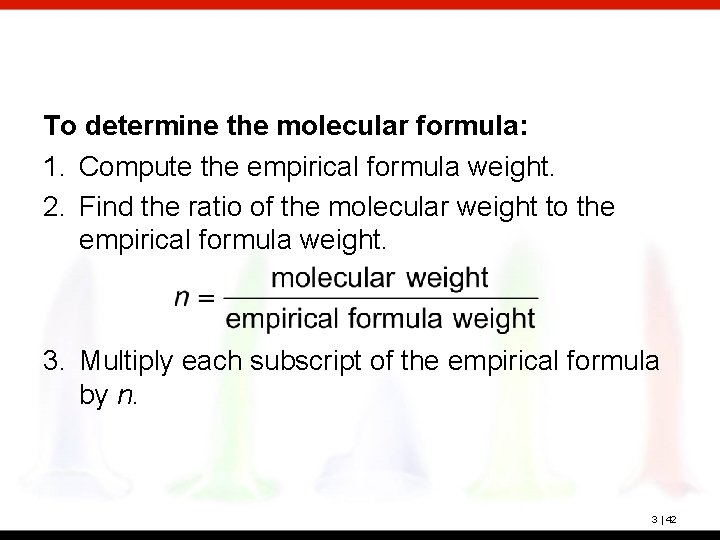To determine the molecular formula: 1. Compute the empirical formula weight. 2. Find the ratio of the molecular weight to the empirical formula weight. 3. Multiply each subscript of the empirical formula by n. 3 | 42? Benzene has the empirical formula CH. Its molecular weight is 78. 1 amu. What is its molecular formula? Molecular formula C 6 H 6 3 | 43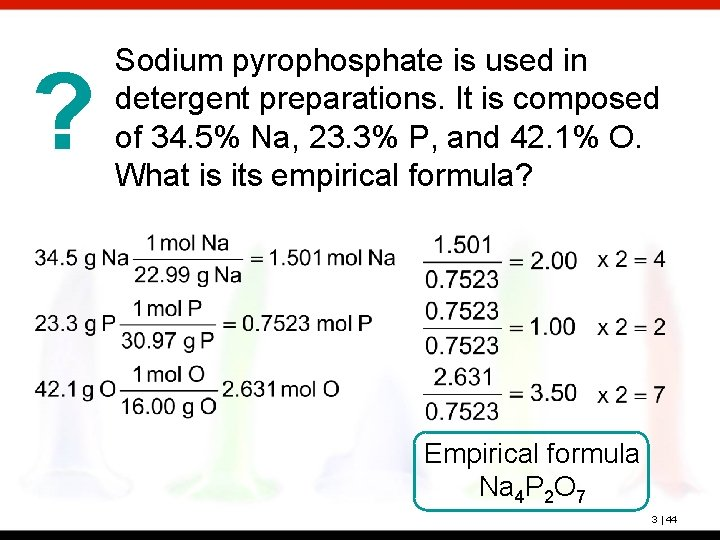? Sodium pyrophosphate is used in detergent preparations. It is composed of 34. 5% Na, 23. 3% P, and 42. 1% O. What is its empirical formula? Empirical formula Na 4 P 2 O 7 3 | 44? Hexamethylene is one of the materials used to produce a type of nylon. It is composed of 62. 1% C, 13. 8% H, and 24. 1% N. Its molecular weight is 116 amu. What is its molecular formula? Empirical formula C 3 H 8 N 3 | 45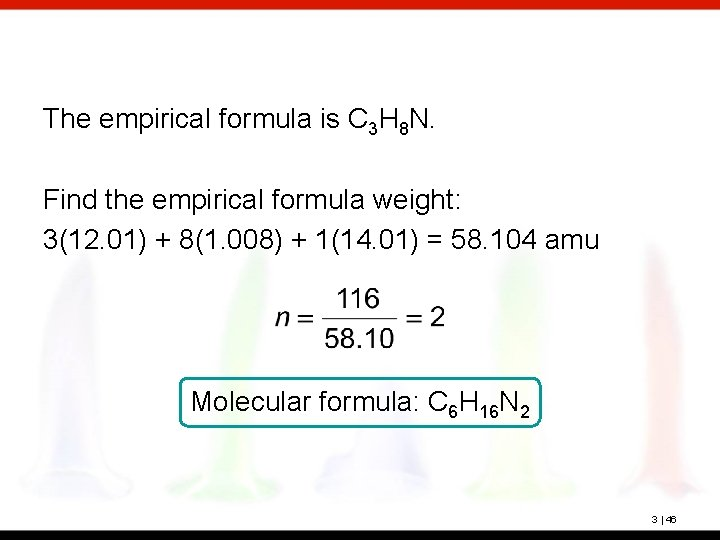The empirical formula is C 3 H 8 N. Find the empirical formula weight: 3(12. 01) + 8(1. 008) + 1(14. 01) = 58. 104 amu Molecular formula: C 6 H 16 N 2 3 | 46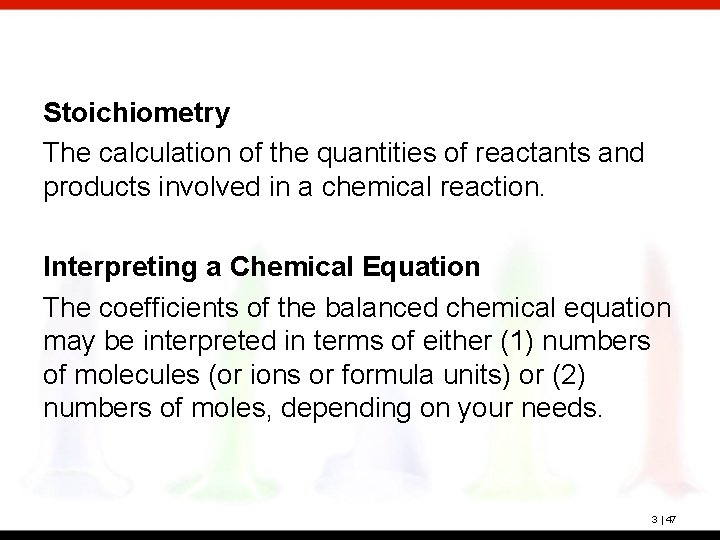Stoichiometry The calculation of the quantities of reactants and products involved in a chemical reaction. Interpreting a Chemical Equation The coefficients of the balanced chemical equation may be interpreted in terms of either (1) numbers of molecules (or ions or formula units) or (2) numbers of moles, depending on your needs. 3 | 47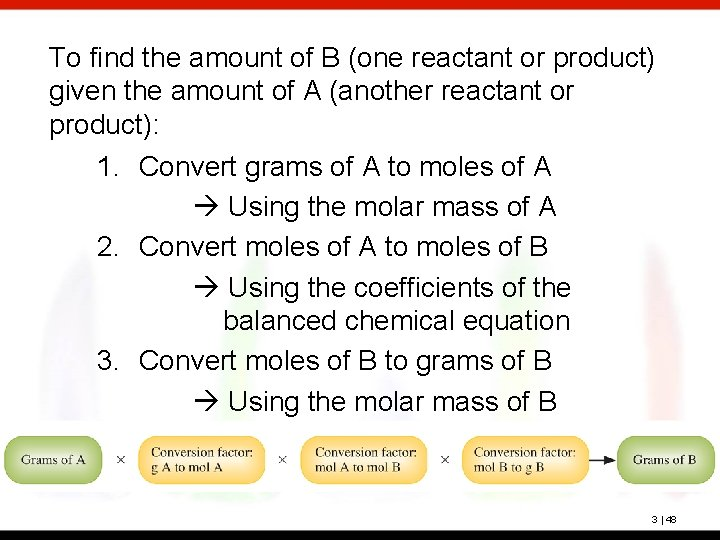To find the amount of B (one reactant or product) given the amount of A (another reactant or product): 1. Convert grams of A to moles of A Using the molar mass of A 2. Convert moles of A to moles of B Using the coefficients of the balanced chemical equation 3. Convert moles of B to grams of B Using the molar mass of B 3 | 48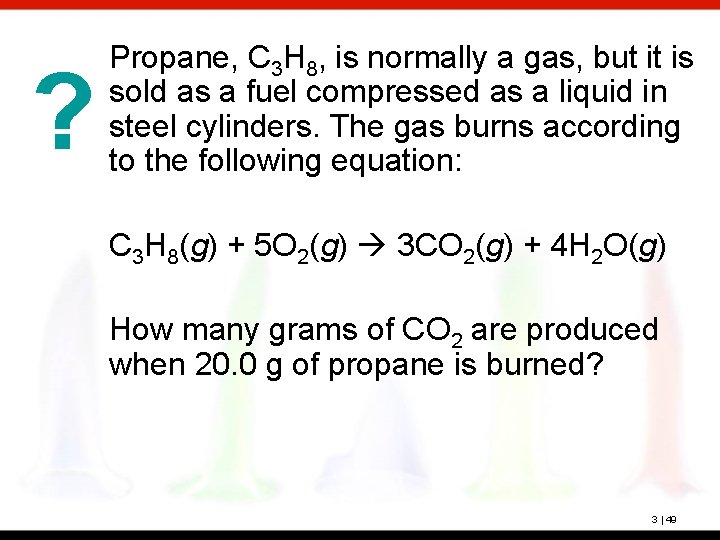? Propane, C 3 H 8, is normally a gas, but it is sold as a fuel compressed as a liquid in steel cylinders. The gas burns according to the following equation: C 3 H 8(g) + 5 O 2(g) 3 CO 2(g) + 4 H 2 O(g) How many grams of CO 2 are produced when 20. 0 g of propane is burned? 3 | 49Molar masses C 3 H 8: 3(12. 01) + 8(1. 008) = 44. 094 g CO 2: 1(12. 01) + 2(16. 00) = 44. 01 g 59. 9 g CO 2 (3 significant figures) 3 | 50? Propane, C 3 H 8, is normally a gas, but it is sold as a fuel compressed as a liquid in steel cylinders. The gas burns according to the following equation: C 3 H 8(g) + 5 O 2(g) 3 CO 2(g) + 4 H 2 O(g) How many grams of O 2 are required to burn 20. 0 g of propane? 3 | 51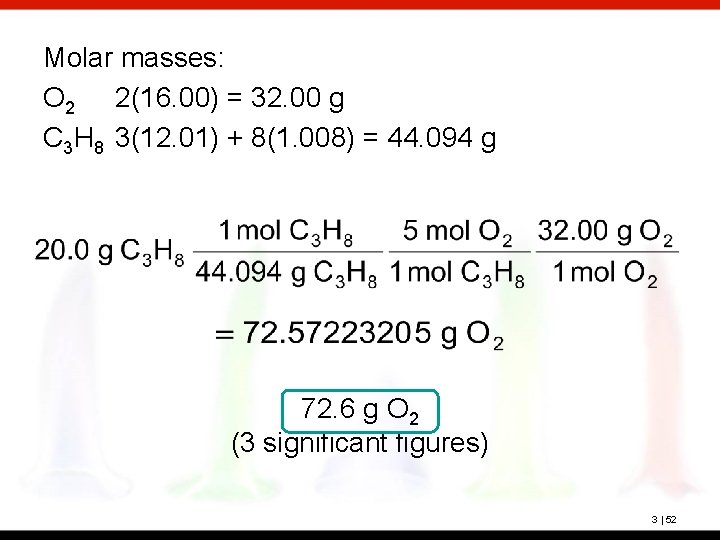Molar masses: O 2 2(16. 00) = 32. 00 g C 3 H 8 3(12. 01) + 8(1. 008) = 44. 094 g 72. 6 g O 2 (3 significant figures) 3 | 52Limiting Reactant The reactant that is entirely consumed when a reaction goes to completion. Once one reactant has been completely consumed, the reaction stops. Any problem giving the starting amount for more than one reactant is a limiting reactant problem. 3 | 53All amounts produced and reacted are determined by the limiting reactant. How can we determine the limiting reactant? 1. Use each given amount to calculate the amount of product produced. 2. The limiting reactant will produce the lesser or least amount of product. 3 | 54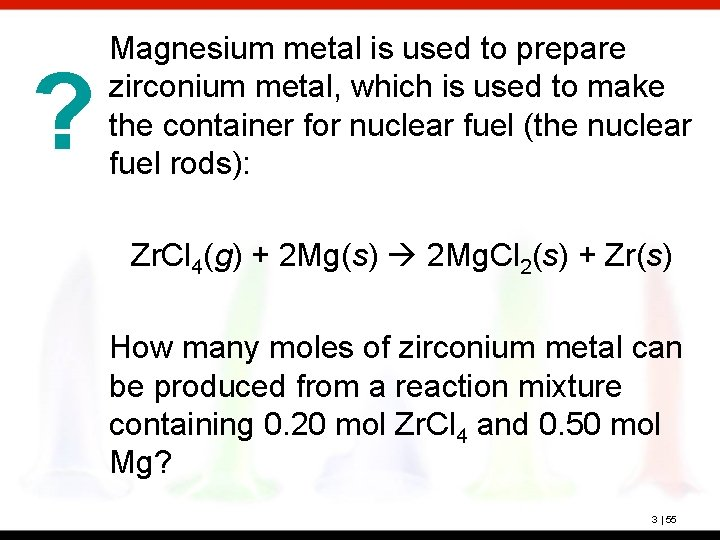? Magnesium metal is used to prepare zirconium metal, which is used to make the container for nuclear fuel (the nuclear fuel rods): Zr. Cl 4(g) + 2 Mg(s) 2 Mg. Cl 2(s) + Zr(s) How many moles of zirconium metal can be produced from a reaction mixture containing 0. 20 mol Zr. Cl 4 and 0. 50 mol Mg? 3 | 55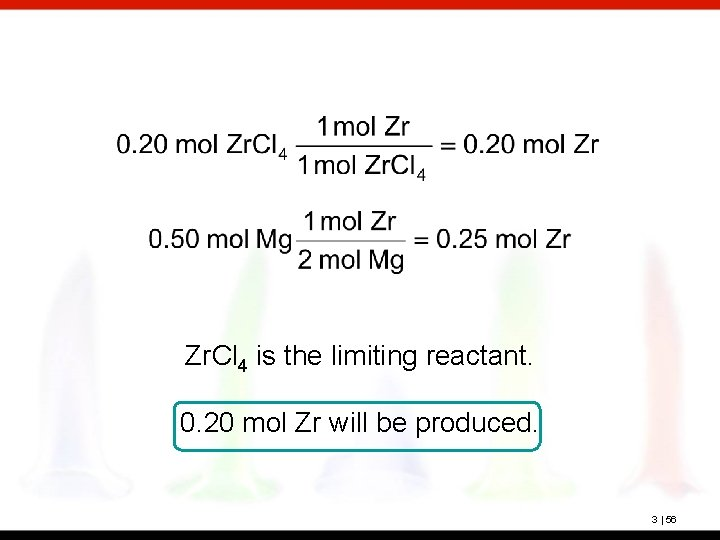Zr. Cl 4 is the limiting reactant. 0. 20 mol Zr will be produced. 3 | 56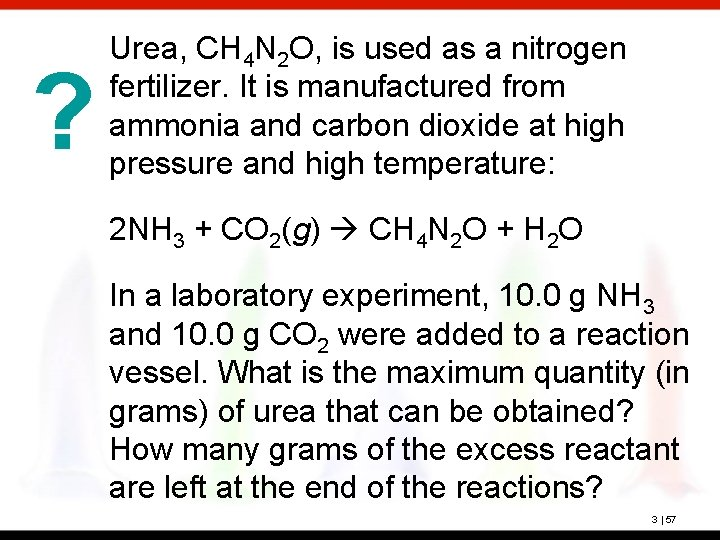? Urea, CH 4 N 2 O, is used as a nitrogen fertilizer. It is manufactured from ammonia and carbon dioxide at high pressure and high temperature: 2 NH 3 + CO 2(g) CH 4 N 2 O + H 2 O In a laboratory experiment, 10. 0 g NH 3 and 10. 0 g CO 2 were added to a reaction vessel. What is the maximum quantity (in grams) of urea that can be obtained? How many grams of the excess reactant are left at the end of the reactions? 3 | 57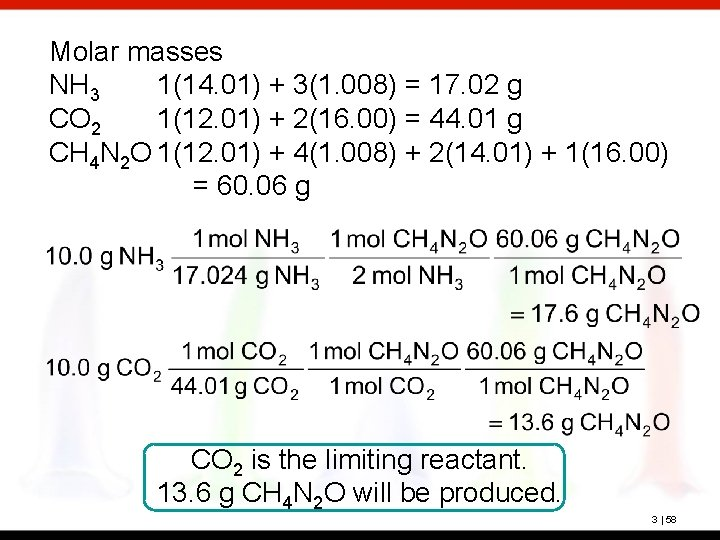Molar masses NH 3 1(14. 01) + 3(1. 008) = 17. 02 g CO 2 1(12. 01) + 2(16. 00) = 44. 01 g CH 4 N 2 O 1(12. 01) + 4(1. 008) + 2(14. 01) + 1(16. 00) = 60. 06 g CO 2 is the limiting reactant. 13. 6 g CH 4 N 2 O will be produced. 3 | 58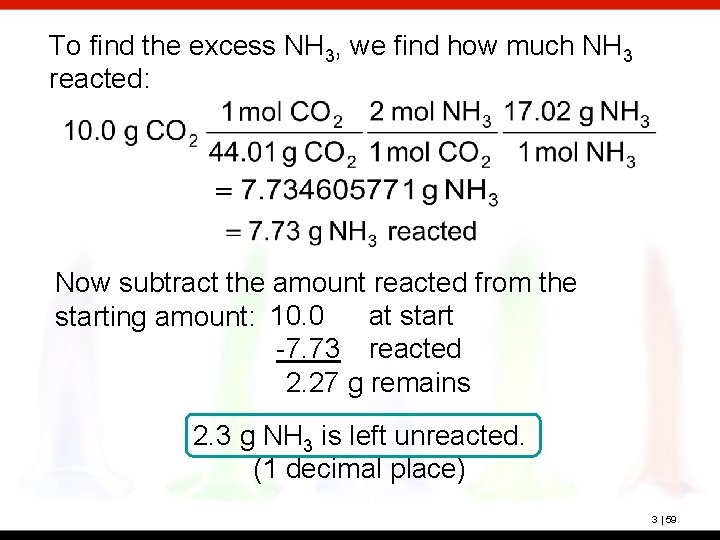To find the excess NH 3, we find how much NH 3 reacted: Now subtract the amount reacted from the at starting amount: 10. 0 -7. 73 reacted 2. 27 g remains 2. 3 g NH 3 is left unreacted. (1 decimal place) 3 | 59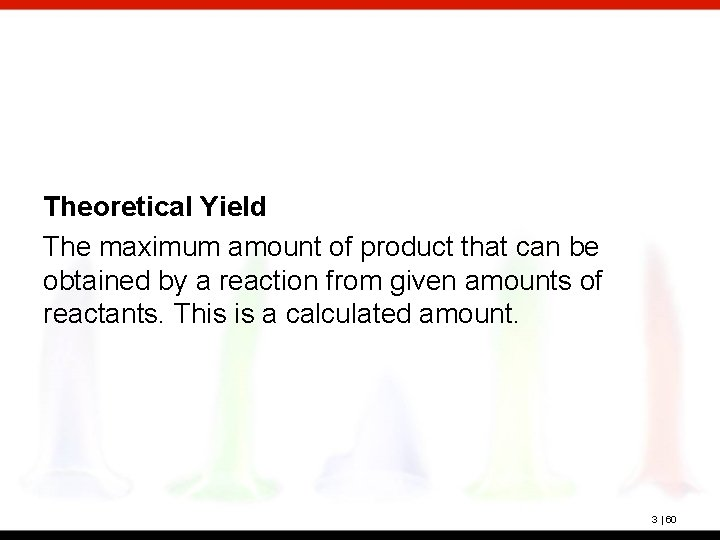Theoretical Yield The maximum amount of product that can be obtained by a reaction from given amounts of reactants. This is a calculated amount. 3 | 60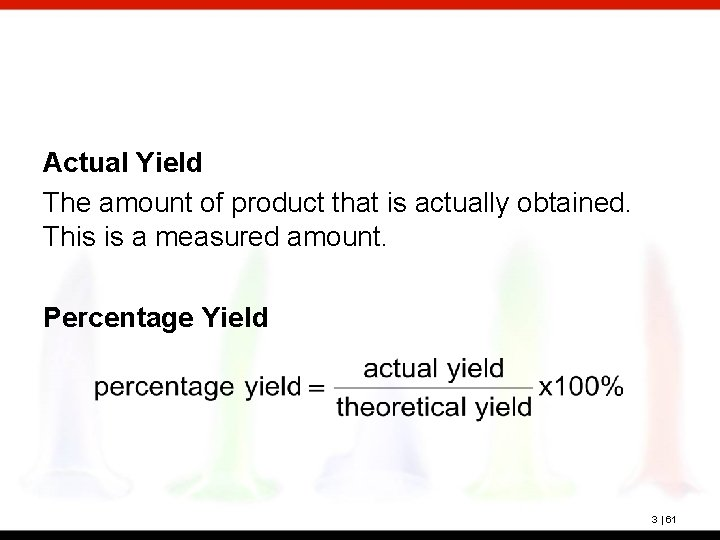Actual Yield The amount of product that is actually obtained. This is a measured amount. Percentage Yield 3 | 61? 2 NH 3 + CO 2(g) CH 4 N 2 O + H 2 O When 10. 0 g NH 3 and 10. 0 g CO 2 are added to a reaction vessel, the limiting reactant is CO 2. The theoretical yield is 13. 6 of urea. When this reaction was carried out, 9. 3 g of urea was obtained. What is the percent yield? Theoretical yield = 13. 6 g Actual yield = 9. 3 g = 68% yield (2 significant figures) 3 | 62